Courses
Courses for Kids
Free study material
Free LIVE classes
More

# NCERT Solutions for Class 6 Maths Chapter 9 - ExerciseLIVE
Join Vedantu’s FREE Mastercalss

## NCERT Solutions for Class 6 Maths Chapter 9 Data Handling (Ex 9.2) Exercise 9.2

Free PDF download of NCERT Solutions for Class 6 Maths Chapter 9 Exercise 9.2 (Ex 9.2) and all chapter exercises at one place prepared by expert teacher as per NCERT (CBSE) books guidelines. Class 6 Maths Chapter 9 Data Handling Exercise 9.2 Questions with Solutions to help you to revise complete Syllabus and Score More marks. Register and get all exercise solutions in your emails.

Last updated date: 01st Oct 2023
Total views: 508.8k
Views today: 7.08k

## Access NCERT Solutions for Class 6 Mathematics Chapter 9- Data Handling

Exercise 9.2

1. Total number of animals in five villages is as follows:

Village A: $80$ $\qquad\qquad$ Village B: $120$

Village C:  $90$ $\qquad\qquad$ Village D: $40$

Village E:  $60$

Prepare a pictograph of these animals using one symbol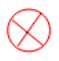to represent $10$ animals and answer the following questions:

Ans: Now we will make a table to represent the data. For village A, draw $8$ symbols. For village B, draw $12$ symbols. For village C draw, $9$ symbols. For village D, draw $4$ symbols. For village E, draw $6$ symbols.

 Village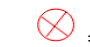= 10 animals Village A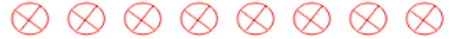$80$ Village B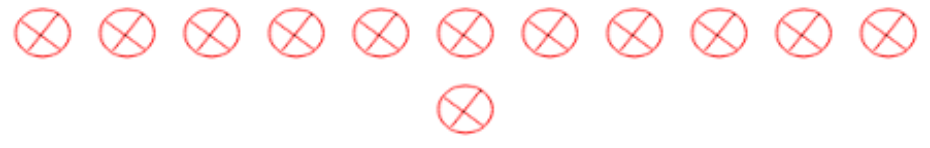$120$ Village C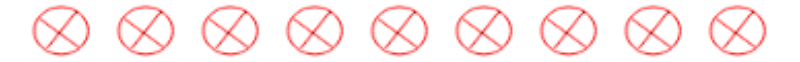$90$ Village D$40$ Village E$60$

(a) How many symbols represent animals of village E?

Ans: Animals of village E are represented by $6$ symbols.

(b) Which village has the maximum number of animals?

Ans: Village B has a maximum number of animals.

(c) Which village has more animals: village A or village C?

Ans: Since $90 > 80$ so, village C has more animals.

2. Total number of students of a school in different years is shown in the following table:

Years       $\qquad\qquad$    Number of Students

$1996$  $\qquad\qquad\qquad$          $400$

$1998$   $\qquad\qquad\qquad$         $535$

$2000$    $\qquad\qquad\qquad$        $472$

$2002$    $\qquad\qquad\qquad$        $600$

$2004$    $\qquad\qquad\qquad$        $623$

A. Prepare a pictograph of students using one symbolto represent $100$ students and answer the following questions:

Ans: Now we will make a table to represent the data.

 Years= 100 students $1996$$1998$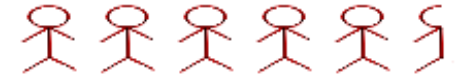$2000$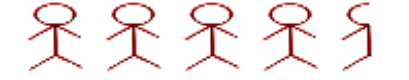$2002$$2004$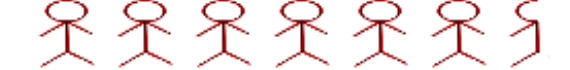(a) How many symbols represent the total number of students in the year $2002$?

Ans: Total number of students in $2002$ is represented by $6$ symbols.

(b) How many symbols represent the total number of students for the year $1998$?

Ans: Total number of students in $1998$ is represented by $5$ complete and $1$ incomplete symbol.

B. Prepare another pictograph of students using any other symbol each representing $50$ students. Which pictograph do you find more informative?

Ans: Now we will make a table to represent the data.

 Years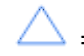= 50 students $1996$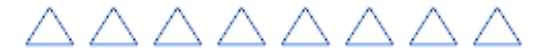$1998$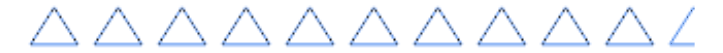$2000$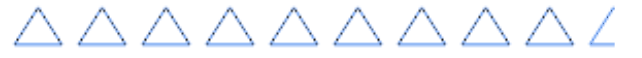$2002$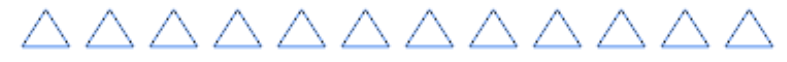$2004$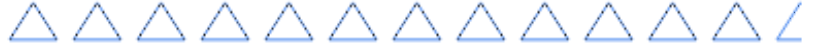This Pictograph is more informative since it tells the closest value of the number of students.

## NCERT Solutions for Class 6 Maths Chapter 9 Data Handling Exercise 9.2

Opting for the NCERT solutions for Ex 9.2 Class 6 Maths is considered as the best option for the CBSE students when it comes to exam preparation. This chapter consists of many exercises. Out of which we have provided the Exercise 9.2 Class 6 Maths NCERT solutions on this page in PDF format. You can download this solution as per your convenience or you can study it directly from our website/ app online.

Vedantu in-house subject matter experts have solved the problems/ questions from the exercise with the utmost care and by following all the guidelines by CBSE. Class 6 students who are thorough with all the concepts from the Subject Data Handling textbook and quite well-versed with all the problems from the exercises given in it, then any student can easily score the highest possible marks in the final exam. With the help of this Class 6 Maths Chapter 9 Exercise 9.2 solutions, students can easily understand the pattern of questions that can be asked in the exam from this chapter and also learn the marks weightage of the chapter. So that they can prepare themselves accordingly for the final exam.

Besides these NCERT solutions for Class 6 Maths Chapter 9 Exercise 9.2, there are plenty of exercises in this chapter which contain innumerable questions as well. All these questions are solved/answered by our in-house subject experts as mentioned earlier. Hence all of these are bound to be of superior quality and anyone can refer to these during the time of exam preparation. In order to score the best possible marks in the class, it is really important to understand all the concepts of the textbooks and solve the problems from the exercises given next to it.

Do not delay any more. Download the NCERT solutions for Class 6 Maths Chapter 9 Exercise 9.2 from Vedantu website now for better exam preparation. If you have the Vedantu app in your phone, you can download the same through the app as well. The best part of these solutions is these can be accessed both online and offline as well.